# 论文阅读 - Learning Feature Pyramids for Human Pose Estimation

PyraNet - Code-Torch

Pyramid Residual Module(PRMs) —— 金字塔残差模块，来增强 DCNNs 的尺度不变性(invariance in scales)；

• Hourglass 网络，conv-deconv 结构；
• 新的权重初始化方法，对 multi-branch 网络权重进行初始化.

• PRMs，学习卷积 filter，建立特征金字塔；
• 给定输入 features，PRMs 采用 multi-branch 网络基于不同采样率进行下采样，以获得不同尺度的特征；
• 然后，对不同尺度的特征学习卷积 filters；
• 再对 filtered 特征下采样到相同分辨率，并相加不同尺度特征.

## 1. Stacked Hourglass Network

[[论文阅读 - Stacked Hourglass Networks for Human Pose Estimation]](http://www.aiuai.cn/aifarm169.html)

Hourglass 网络以 feed-forward 方式学习每个 scale 的信息.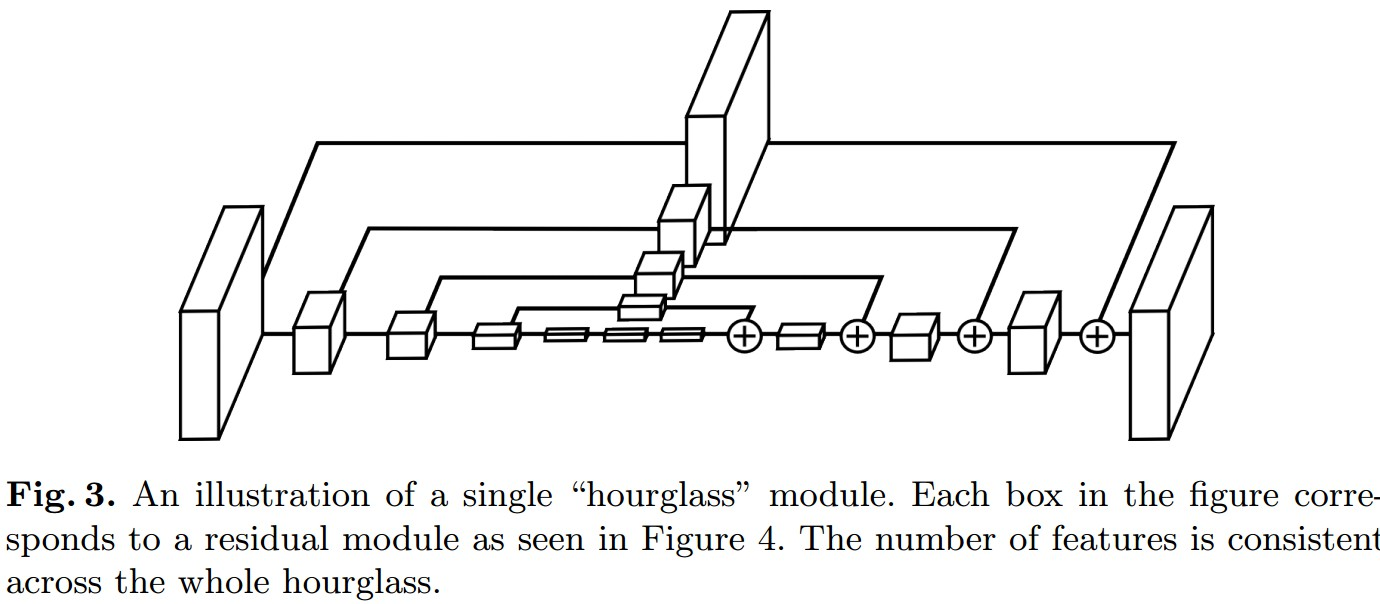Figure 1. single "hourglass" 模块例示. 每一个 box 对应一个 residual 模块.

Residual Unit: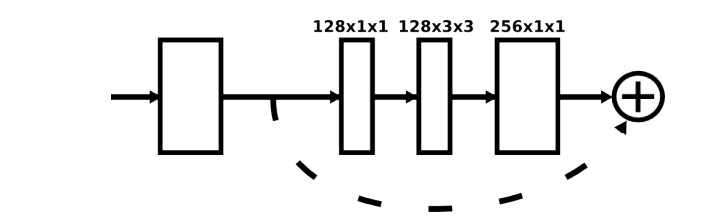Stacked Hourglass Network 训练的中间监督处理：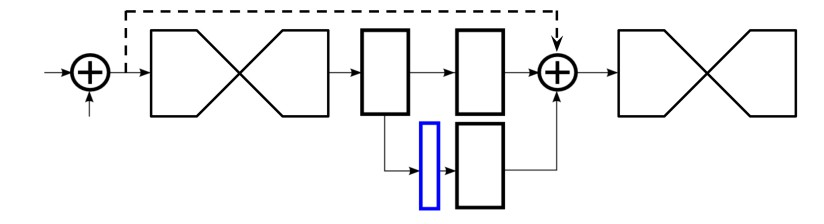Figure 1.1 中间监督处理. 网络输出 heatmaps(蓝色框) ，其后添加训练 loss. 采用 1×1 卷积将 heatmaps 来匹配 intermediate 特征的 channels 数.

## 2 Pyramid Residual Modules(PRMs)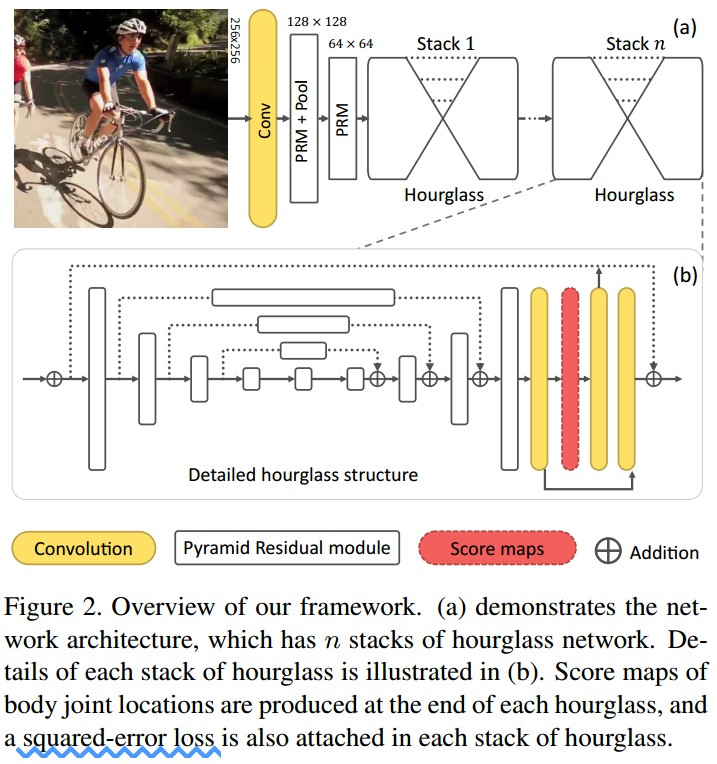Figure 2. 框架. (a) 网络结构，有 n 个 stacks hourglass 网络. (b) 每个 hourglass stack 的细节. 每个 hourglass 的末尾产生 joint 位置的 scoremaps，并使用 squared-error loss.

PRM 学习输入 features 的不同分辨率的 filters.

PRM 表示为：

${ \mathbf{x}^{(l+1)} = \mathbf{x}^{(l)} + \mathcal{P}(\mathbf{x}^{(l)}; \mathbf{W}^{(l)}) }$

${ \mathcal{P}(\mathbf{x}^{(l)}; \mathbf{W}^{(l)}) = g (\sum_{c=1}^{C} f_c(\mathbf{x}^{(l)}; \mathbf{w}_{f_c}^{(l)}); \mathbf{w}_{g}^{(l)}) + f_0(\mathbf{x}^{(l)}; \mathbf{w}_{f_0}^{(l)}) }$

C - pyramid 层的数量；
${ f_c(\cdot) }$ - 第 c 层 pyramid 层的变换；
${ \mathbf{W}^{(l)} = \lbrace \mathbf{w}_{f_c}^{(l)}, \mathbf{w}_{g}^{(l)} \rbrace_{c=0}^{C} }$ - 参数

${ f_c(\cdot) }$ 变换的输出相加，再采用 fliter ${ g(\cdot) }$ 卷积.

PRM 例示如 Figure 3. 每个 ${ f_c(\cdot) }$ 设计为 bottleneck 结构，以降低计算和空间复杂度. 例如， Figure 3 中，采用 1×1 卷积来降低特征维度；然后采用 3×3 卷积对下采样的输入特征集计算新特征；最后，将所有的新特征上采样到相同维度，并相加在一起.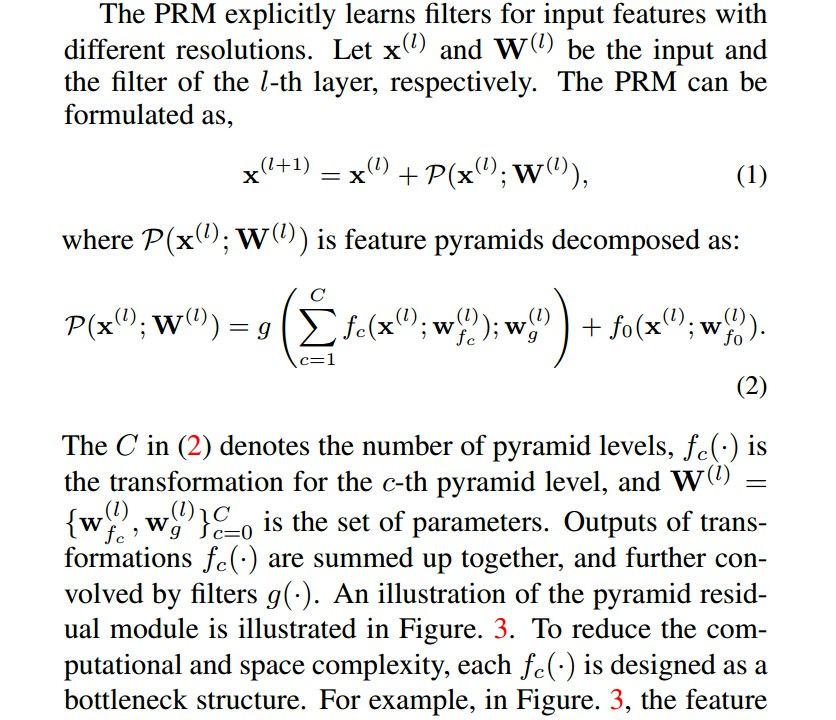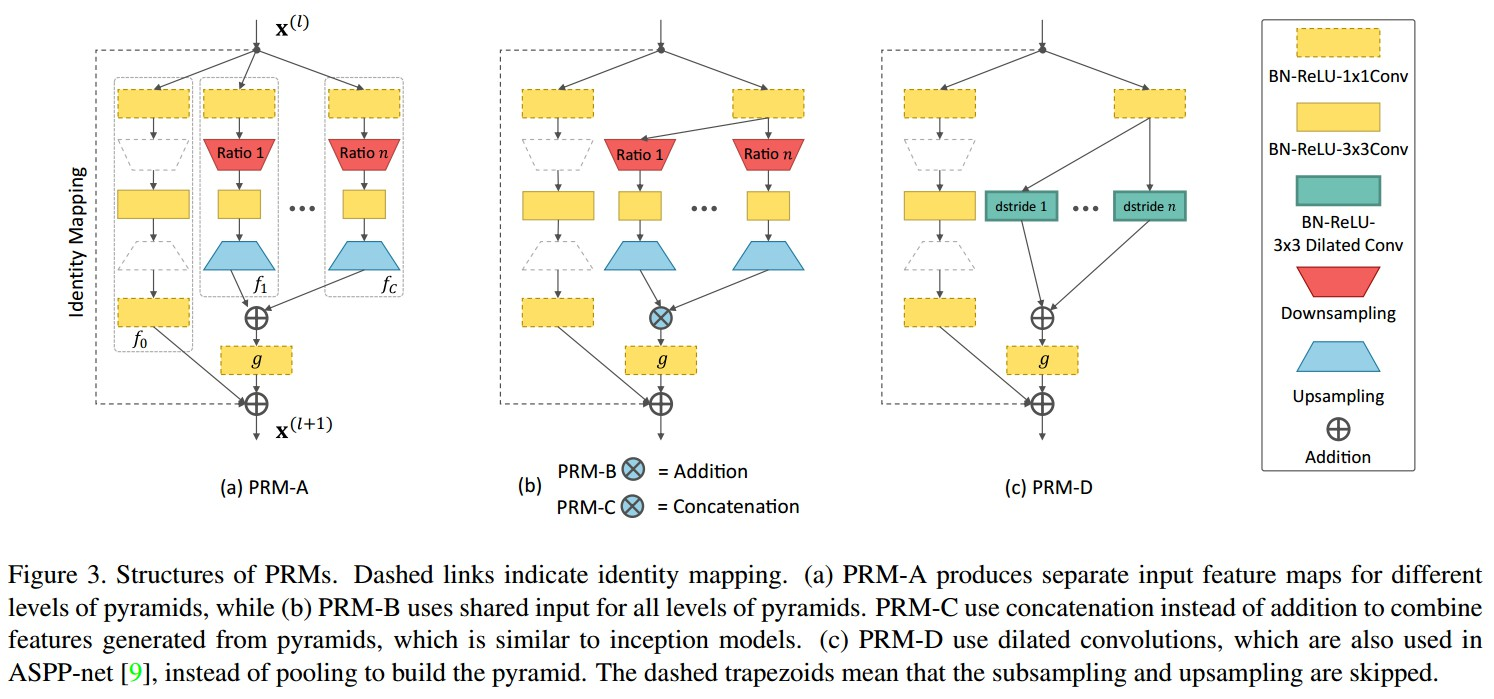Figure 3. PRMs 结构. 虚线表示恒等映射(identity mapping). (a) PRM-A， 将输入 feature maps 从不同金字塔层独立分离；(b) PRM-B，对于所有的金字塔层采用共享输入；PRM-C 采用 concatenation 取代 addition 来组合从金字塔层得到的特征，类似于 inception 模型；(c) PRM-D，利用 dilated 卷积，类似于 ASPP-net，而不是采用 pooling 来构建金字塔. 虚线梯形表示跳过下采样和上采样.

### 2.1 生成输入特征的金字塔

DCNNs一般应用 max-pooling 和 average-pooling 来降低 feature maps 的分辨率，编码其平移不变性.

${ s_c = 2 ^{-M \frac{c}{C}}, c = 0,...,C, M \geq 1 }$

### 2.2 讨论

 - PRM 可以用于 CNN 结构的基础模块，如 stacked hourglass networks-姿态估计， Wide Residual Nets-图像分类，ResNeXt-图像分类.

 - Pyramid 结构的变形：

• 如 Figure 3(a-b)，采用 max-pooling，convolution 和 upsampling 学习特征金字塔；
• 如 Figure 3(c)，PRM-D，采用 dilated convolution 来计算特征金字塔；
• 如 Figure 3(b)，PRM-C，金字塔不同层次特征除了采用相加(summation)处理，还可以采用 concatenation；
• Figure 3(b)，PRM-B 具有相当的表现，但参数相对较少，计算复杂度较低. 【提供的[[Code-Torch]](https://github.com/bearpaw/PyraNet) 应该是基于 PRM-B 模型.】

 - 权重共享：

 - 复杂度

stacked hourglass network 的 residual unit 的输入和输出是 256-d，在residual unit 降低到 128-d.

## 3 网络训练和推断

### 3.1 训练

 - 采用 score maps 来表示关节点位置：

${ \mathbf{z}_k = (x_k, y_k) }$ 为图像中第 k 个关节点的位置.

groundtruth score map ${ \mathbf{S}_k }$ 是均值 ${ \mathbf{z}_k }$ 和方差 ${ \Sigma }$ 的 Gaussian 分布：

${ \mathbf{S}_k(\mathbf{p}) \sim \mathcal{N} (\mathbf{z}_k, \Sigma) }$

${ \mathbf{p} \in R^2 }$ -关节点位置；

${ \Sigma }$ - 单位矩阵 identity matrix ${ \mathbf{I} }$ .

 - Loss 函数：

${ \mathcal{L} = \frac{1}{2} \sum_{n=1}^{N} \sum _{k=1}^{K} ||\mathbf{S}_k- \hat{\mathbf{S}}_k||^2 }$

N - 样本数

### 3.2 推断

${ \hat{\mathbf{z}}_k = arg max _{\mathbf{p}} \hat{\mathbf{S}}_k(\mathbf{p}), k=1,...,K }$

### 3.3 网络初始化方法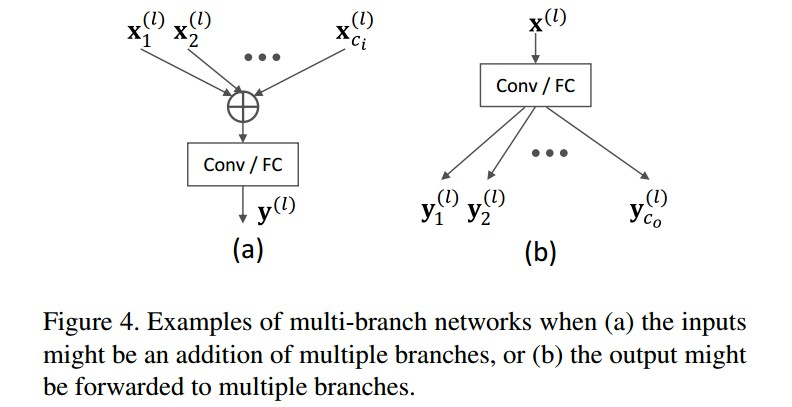### 3.4 Output Variance Accumulation 输出方差的累积问题

• 恒等映射 Identity mapping 会导致随着网络的加深，响应的变化逐渐增加，使得优化难度增大.
• 当两个 residual units 的输出相加时，这里采用 batch_normalization 和 ReLU 后接 $1×1$ 的卷积来代替恒等映射. 如 Figure 6.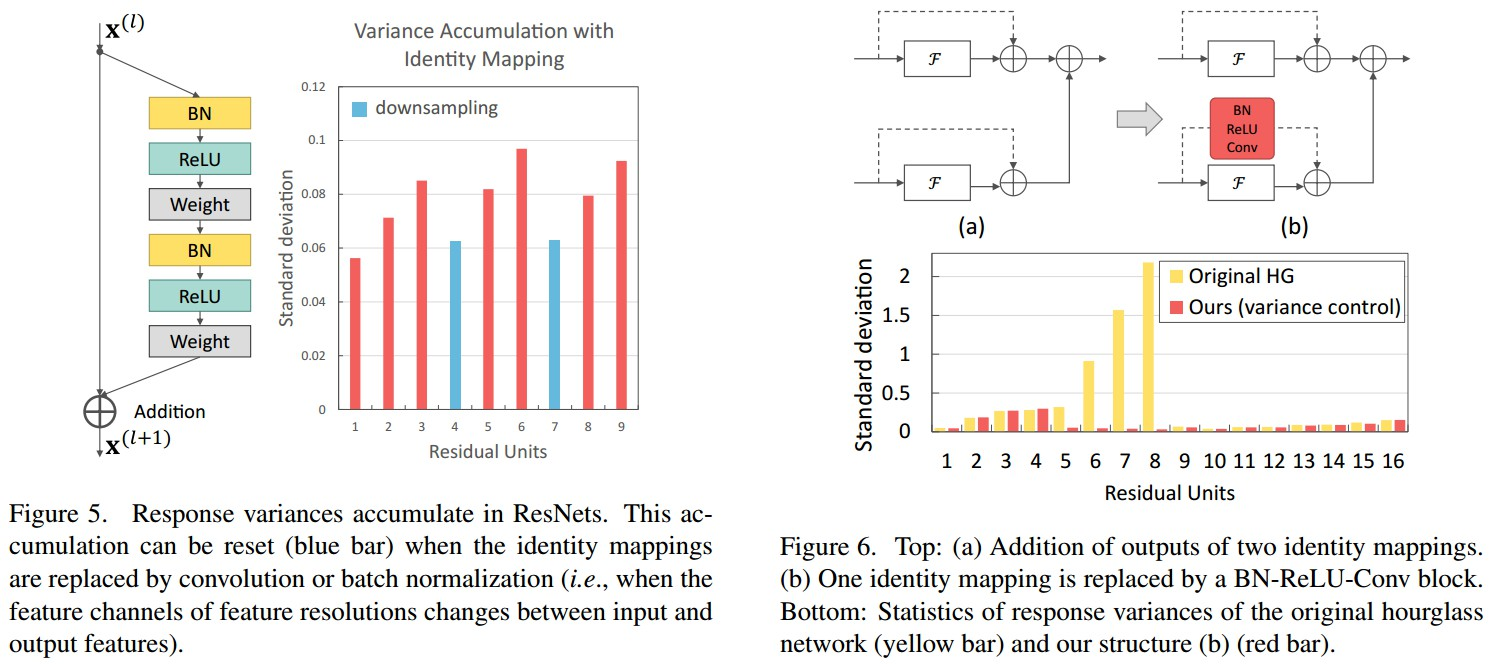## 4 实现和结果

### 4.1 实现细节

• 输入图片根据标注的人体位置和 scale ，从 resized 图片中裁剪尺寸 256×256.
• LSP test 集：直接采用图像中心作为人体位置，根据图片尺寸估计人体 scale；
• 训练数据增广：scaling，rotation，flipping，color noise等；
• 采用 Torch 训练
• 网络优化方法为 RMSProp，4 Titan X GPUs，mini-batch size 为 16，每张 GPU 4 张图片，200 epoches.
• 初始化学习率 7 × 10^{-4}，在 150 epoch 和 170 epoch 各减少 10×.
• Testing 对 6-scale 图像 Pyramids 并 flipping 进行.

### 4.2 ResultsMPII 和 LSP 数据集上不同方法对比：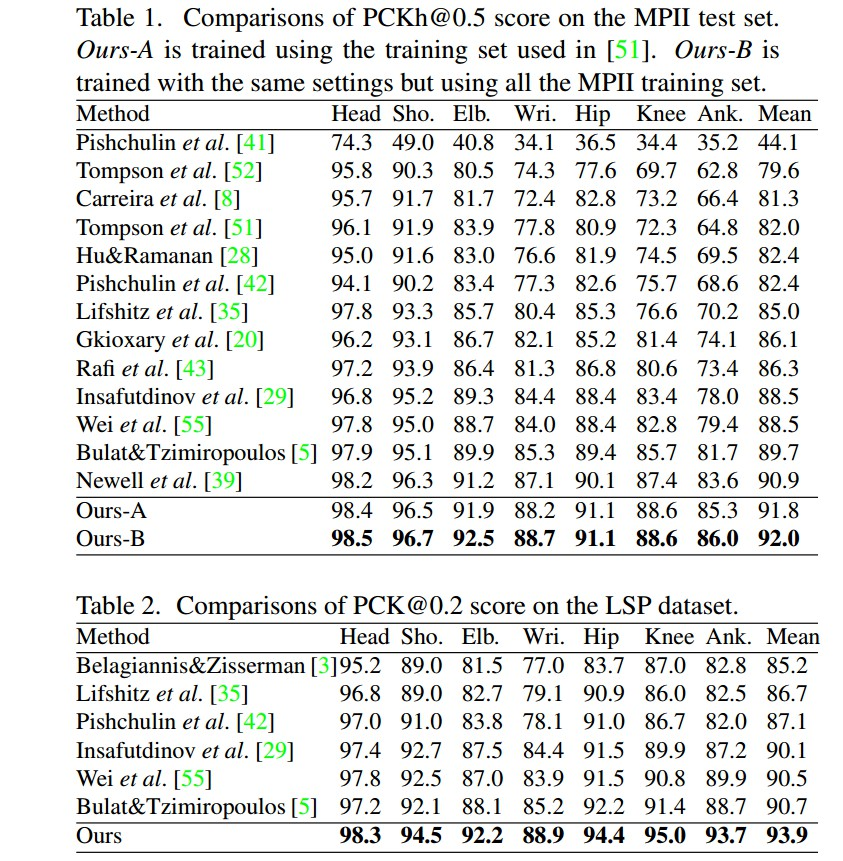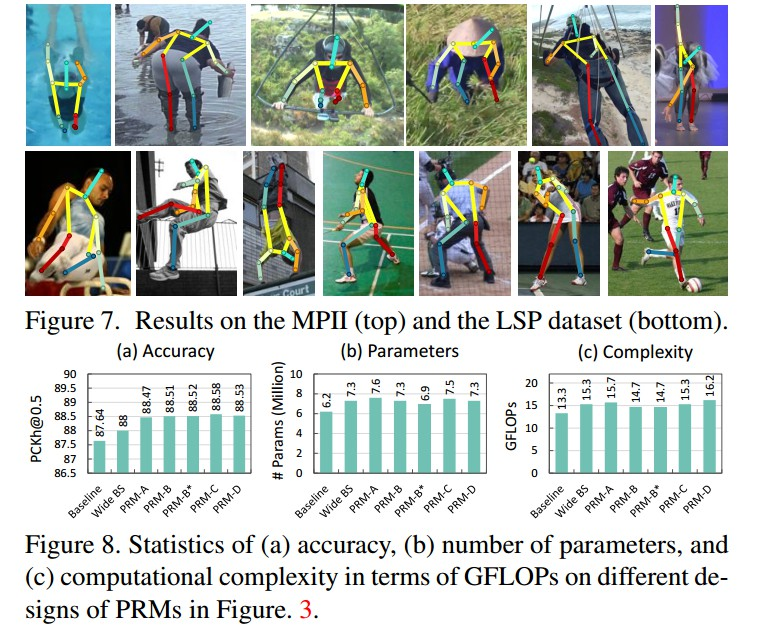Last modification：October 10th, 2018 at 01:05 am